/

### Function Description

Returns optimised transition probabilities as an output array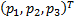given lattice levels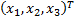which an underlying at the current lattice node can move to, given that we want the sum of the probabilities to add to SumWts (will almost always be 1, as otherwise the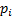are not actually probabilities), the weighted mean of the underlying at the end of this period to be WtdMean and the one-period volatility of the underlying to be WtdStd.

We thus want: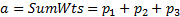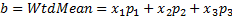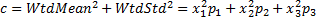Thus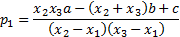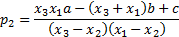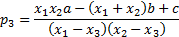Contents | Prev | Next